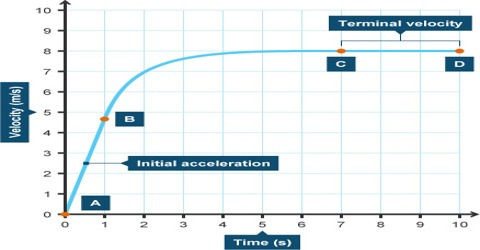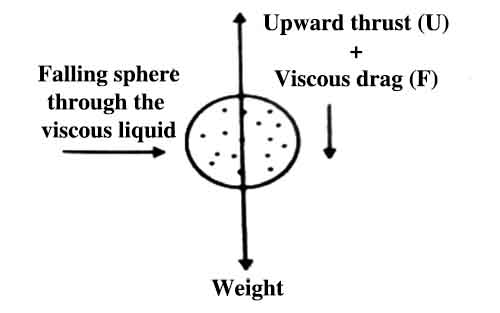# Theoretical Explanation of Terminal Velocity

Theoretical Explanation of Terminal Velocity

From Stokes law – “the force required to move a sphere through a given viscous fluid at a low uniform velocity is directly proportional to the velocity and radius of the sphere”; it is sent that the retarding force on a body is equal to the velocity of the body. If v = 0, then F = 0. Again, if v increases F also increases. From this it can be said that when a sphere falls due to gravitational force through a fluid, initially a velocity increases for acceleration due to gravity and simultaneously retarding or opposing force, on it also increases. As a result the net a resultant acceleration starts decreasing [figure]. At one stage, resultant acceleration becomes zero. Then the body continues to fall with a constant velocity. This constant velocity is called the terminal velocity.If a metallic sphere is allowed to fall through a viscous fluid the downward force i.e., weight is greater than upward force U + F. Here U is upward force i.e., buoyancy and F = upward resistive i.e., viscous force. As a result the sphere acquires downward acceleration. Then the sphere continues to move downward. No resultant force acts on it, so the velocity reaches to a maximum constant value. This is the terminal velocity v, ρf and ρs are respectively densities of the fluid and the sphere and if η is the coefficient of viscosity, then terminal velocity can be determined in the following way.

Equation of Stokes terminal velocity:

Let the density of the material of the sphere be ρ and density of the medium be σ, then gravitational force on the sphere is,

F = weight of the body = mass x acceleration due to gravity = volume x density x acceleration due to gravity.

= V x ρ x g = 4/3 πr2 ρg    (here, V = volume of the sphere)

According to the law of Archimedes, weight lost by the sphere = upward force applied by the medium = 4/3 πr2σg

So, effective downward force or effective weight of the sphere,

F = 4/3 πr2ρg – πr2σg

= 4/3 πr2(ρ – σ)g

When upward force due to viscosity and the effective weight of the sphere will be equal, then the body will start falling at terminal velocity.

We get, 6πηrv = 4/3 πr2(ρ – σ)g, F = viscous force = 6πηrv (Stokes law)

or, v = [(2r2/9) (ρ – σ)g / η]

This is the equation for the stokes terminal velocity.